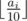Description

Petya loves computer games. Finally a game that he’s been waiting for so long came out!

The main character of this game has n different skills, each of which is characterized by an integer ai from 0 to 100. The higher the number ai is, the higher is the i-th skill of the character. The total rating of the character is calculated as the sum of the values ​​offor all i from 1 to n. The expression ⌊ x⌋ denotes the result of rounding the number x down to the nearest integer.

At the beginning of the game Petya got k improvement units as a bonus that he can use to increase the skills of his character and his total rating. One improvement unit can increase any skill of Petya’s character by exactly one. For example, if a4 = 46, after using one imporvement unit to this skill, it becomes equal to 47. A hero’s skill cannot rise higher more than 100. Thus, it is permissible that some of the units will remain unused.

Your task is to determine the optimal way of using the improvement units so as to maximize the overall rating of the character. It is not necessary to use all the improvement units.

Input

The first line of the input contains two positive integers n and k(1 ≤ n ≤ 105,0 ≤ k ≤ 107) — the number of skills of the character and the number of units of improvements at Petya’s disposal.

The second line of the input contains a sequence of n integers ai (0 ≤ ai ≤ 100), where ai characterizes the level of the i-th skill of the character.

Output

The first line of the output should contain a single non-negative integer — the maximum total rating of the character that Petya can get using k or less improvement units.

Examples

input

2 4
7 9

output

2

input

3 8
17 15 19

output

5

input

2 2
99 100

output

20

Solution

1. 先将人物初始等级统计出来。
2. b[i]=a[i] mod 10
3. 对 b[i] 进行升序排序,从高到低贪心求解。
4. k−10+b[i]>=0 ,则说明还可以继续加技能，继续向后执行。
5. 人物最高等级为 n∗10 ,最终人物等级不能超过n∗10 .

  1 2 3 4 5 6 7 8 9 10 11 12 13 14 15 16 17 18 19 20 21 22 23 24 25 26 27 28 29 30 31 32 33 34 35 36 37  #include #include #include #include using namespace std; #define N 11111111 int a[N], b[N]; int main( ) { int n, k; while (~scanf("%d%d", &n, &k)) { int ans = 0; for (int i = 0; i scanf("%d", &a[i]); ans += a[i] / 10; b[i] = a[i] % 10; } sort(b, b + n); for (int i = n - 1; i >= 0; i--) { if (k - 10 + b[i] >= 0) { ans++; k = k - 10 + b[i]; if (k >= 10 && i == 0) ans += k / 10; if (ans >= 10 * n) ans = 10 * n; } else break; } printf("%d\n", ans); } return 0; }